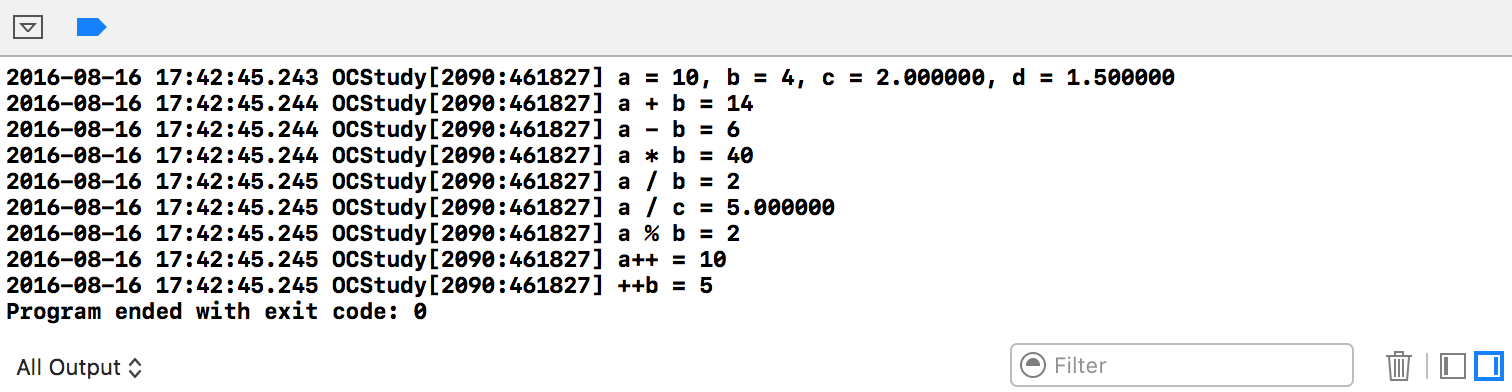# OC算数表达式：1-基本运算符

Objective-C语言是C语言的基础上发展起来的，所以沿用了C语言的运算规则。Objective-C语言的运算符不仅具有不同的优先级，而且还有一个特点，就是它的结合性。在表达式中，各运算量参与运算的先后顺序不仅要遵守运算符优先级别的规定，还要受运算符结合性的制约，以便确定是自左向右进行运算，还是自右向左进行运算。这种结合性是其它高级语言的运算符所没有的，因此也增加了Objective-C语言的复杂性。

# 基本概念

• 算术表达式：用算术运算符和括号将运算对象（也称操作数）连接起来的、符合C语法规则的式子。

• 算术运算符: 用于各类数值运算。包括加(+)、减(-)、乘(*)、除(/)、求余(或称模运算，%)、自增(++)、自减(--)共七种。

• 运算符的优先级：Ｃ语言中，运算符的运算优先级共分为15级。1级最高，15级最低。在表达式中，优先级较高的先于优先级较低的进行运算。而在一个运算量两侧的运算符优先级相同时，则按运算符的结合性所规定的结合方向处理。

• 运算符的结合性：Ｃ语言中各运算符的结合性分为两种，即左结合性(自左至右)和右结合性(自右至左)。例如算术运算符的结合性是自左至右，即先左后右。如有表达式x-y+z则y应先与“-”号结合，执行x-y运算，然后再执行+z的运算。这种自左至右的结合方向就称为“左结合性”。而自右至左的结合方向称为“右结合性”。 最典型的右结合性运算符是赋值运算符。如x=y=z,由于“=”的右结合性，应先执行y=z再执行x=(y=z)运算。Ｃ语言运算符中有不少为右结合性，应注意区别，以避免理解错误。

# 基本运算符

• 加法运算符+：加法运算符为双目运算符，即应有两个量参与加法运算。如a+b,4+8等。加法运算符具有左结合性。
• 减法运算符-：减法运算符为双目运算符。但“-”也可作负值运算符，此时为单目运算，如-x,-5等。具有左结合性。
• 乘法运算符*：双目运算，具有左结合性。
• 除法运算符/：双目运算，具有左结合性。注意：使用除法运算符时，当参与运算的变量均为整型时，运算结果也为整型，会舍去小数。
• 求余运算符(模运算符)%：双目运算，具有左结合性。要求参与运算的量均为整型。 求余运算的结果等于两数相除后的余数。
• 自增、自减运算符：自增1运算符记为++，其功能是使变量的值自增1。自减1运算符记为--，其功能是使变量值自减1。自增1，自减1运算符均为单目运算，都具有右结合性。可有以下几种形式：
``````        ++i;    //i自增1后再参与其它运算。
--i;    //i自减1后再参与其它运算。
i++;    //i参与运算后，i的值再自增1.
i--;    //i参与运算后，i的值再自减1。
``````

``````//严禁出现下面风格的代码，这在C标准里，叫做未定义行为，一切未定义行为的执行后果都是不可知的，编译器不会报错，但是会报警告。
int main(int argc, const char * argv[]) {
@autoreleasepool {
int i = 1,j = 1,p,q;
p = (i++) + (i++) + (i++);
q = (++j) + (++j) + (++j);
printf("%d,%d,%d,%d",p,q,i,j);
}
return 0;
}
``````

# 示例代码

``````#import <Foundation/Foundation.h>
int main(int argc, const char * argv[]) {
@autoreleasepool {
int a = 10, b = 4;
float c = 2.0, d = 1.5;
// 打印a, b, c, d的值
NSLog(@"a = %d, b = %d, c = %f, d = %f", a, b, c, d);
// 加法
NSLog(@"a + b = %d", a + b);
// 减法
NSLog(@"a - b = %d", a - b);
// 乘法
NSLog(@"a * b = %d", a * b);
// 除法
NSLog(@"a / b = %d", a / b);    //两个整型相除，结果也为整型，小数部分舍去
NSLog(@"a / c = %f", a / c);    //参与运算的量有浮点型时，运算结果也为浮点型
// 模运算（取余）
NSLog(@"a %% b = %d", a % b);   //NSLog中 %% 表示打印一个 %，模运算要求左右两边均为整型
// 自增（自减类似）
NSLog(@"a++ = %d", a++);    // 先取值，后加1
NSLog(@"++b = %d", ++b);    // 先加1，后取值
}
return 0;
}
``````# 代码下载地址：

https://github.com/99ios/2.2.1